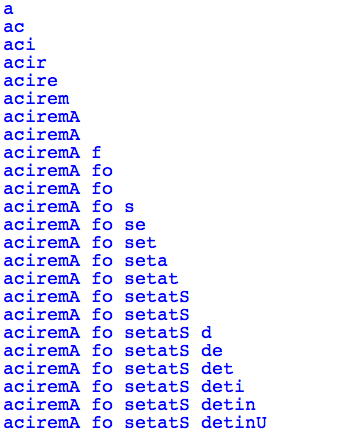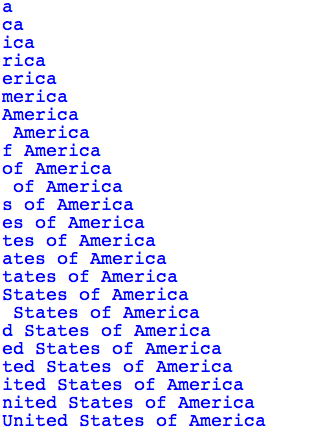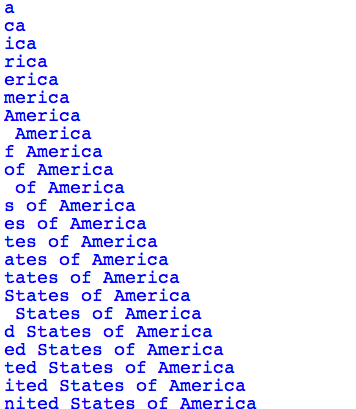# Using a Negative Change Value with Range¶

The `range(start, end, change)` function can have 3 parameters: the `start`, the `end`, and the amount to `change` by. It returns a list that begins with the `start` value and generates the rest of the list items by adding the `change` value to the `start` value. It stops generating the list when the current value is equal or greater than the `end` value. So the generated list includes the `start` value and excludes the `end` value. We have mostly used the `range` function to create an increasing sequence of numbers like [0, 1, 2, 3, 4, 5]. But, it can also be used to create a decreasing sequence like [5, 4, 3, 2, 1, 0] as shown below. Remember that the second number to the range function says when to stop and that number is not included in the returned list of numbers.

Here’s a program we saw earlier, but with the `range` function parameters changed to create a decreasing list from the last valid index (the length of the list minus 1) to 0.

csp-16-7-1: Why do we start at `len(source)-1` in this program?

• If we started with len(source), we would get an error for indexing past the end of the list
• Right -- the end element is at index len(source)-1
• It is a mistake and should be len(source)
• No -- if accessed len(source) as an index, we would be going past the end of the list
• Because we have -1 in the other two spots
• We have -1 in the end position because we want to stop at zero, and we have an increment of -1 (last position)

Can you figure out what the following program does?

```source = "United States of America"
slowly = ""
for index in range(len(source)-1,-1,-1):
slowly = slowly + source[index]
print(slowly)
```

Try to figure out what the program above does, then try to answer this question.

csp-16-7-2: Which one of these is the output of that program?

•• This takes letters from the end of the string forward, and adds them to the end
•• This one is adding up letters in the forward direction
•• This one ends at 0 (or rather, 1)

Write code to count down by 2 from 10 to 0.